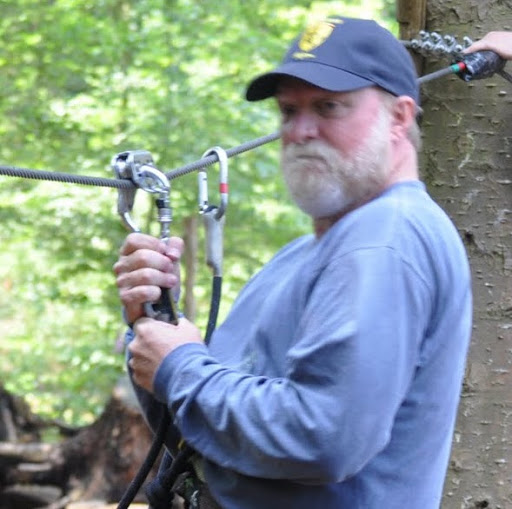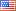Posted in | Quantum Computing

# A New Limit Found for Quantum Computing

Yurchanka Siarhei/shutterstock.com

Researchers at the Skolkovo Institute of Science and Technology, Moscow, have discovered a limit on the ability of quantum computing algorithms to solve certain kinds of problems.

In a paper entitled “Reachability Deficits in Quantum Approximate Optimization”, V. Akshay, H. Philathong, M.E.S. Morales, and J.D. Biamonte study the ability of the Quantum Approximate Optimization Algorithm (QAOA) to solve constraint satisfaction problems. In these problems, values of boolean variables must be found that satisfy several logical constraints.

For three variables, this problem is NP-complete and thus, difficult to solve classically. Since the three-variable problem is NP-complete, problems with more than three variables can always be reduced to the three-variable case.

QAOA tackles this problem by setting up a system of qubits whose quantum state represents the space of possible solutions, and whose energy is proportional to the number of constraints violated. A series of operations is performed to minimize the expected energy of the system, and a measurement is made. If the problem has a logical solution, the energy of the ground state should be zero, whereas a non-zero ground state energy indicates that the problem is unsatisfiable.

Previous work on QAOA had shown that for some problems (such as neural network optimization), it suffers from barren plateaus - where starting states exist from which the correct solution is unlikely to be reached. This is due to the gradient of the Hamiltonian concerning the solution parameters being small in those regions. However, barren plateaus do not occur in constraint satisfaction problems, as they deal with discrete rather than continuous variables.

A key parameter of constraint satisfaction problems is the clause-to-variable ratio, that is the number of constraints that must be satisfied divided by the number of variables. Another key parameter is the circuit depth, which is the number of quantum operations necessary to prepare the starting state of the system before measurement.

Akshay et al. found that not all starting states for a given problem can converge to the ground state. Conversely, for a given starting state, there are constraint satisfaction problems for which the correct solution cannot be found. These conditions are known as reachability deficits, and they become more common as the clause-to-variable ratio increases.

For a given value of the clause-to-variable ratio, there is a critical value of the circuit depth below which the system cannot be guaranteed to converge to the ground state. This critical depth increases with the number of constraints.

While classically there is a critical clause-to-variable ratio for the three-variable case above, in which most problems are unsatisfiable, and classical algorithms slow down near this point, the existence of reachability deficits is not related to the classical behavior.

Furthermore, reachability deficits are even found in the two variable cases, which is classically solvable in polynomial time. This shows that reachability deficits are a feature of the Quantum Approximate Optimization Algorithm itself, and not simply of the constraint satisfaction problem.

The authors have shown that similar behavior occurs in Grover’s Algorithm, a related quantum algorithm that finds the inverse of an arbitrary function. For both problems, two different forms of the driver Hamiltonian, which is used to prepare the initial state of the quantum system, are used.
QAOA is important because it is implementable with current quantum computing hardware and can be applied to a variety of different problems. However, previous work has shown that it does not outperform classical methods for small circuit depths, and the work of Askay et al. shows that large circuit depths are necessary to ensure that the correct solution is reached.

Therefore, while quantum computing has the potential to solve hard problems faster than classical computing, it is necessary to thoroughly understand the limitations of the algorithms to ensure they give correct answers in practice.

## Citations

• APA

Bleackley, Peter. (2019, August 01). A New Limit Found for Quantum Computing. AZoQuantum. Retrieved on August 14, 2020 from https://www.azoquantum.com/News.aspx?newsID=6593.

• MLA

Bleackley, Peter. "A New Limit Found for Quantum Computing". AZoQuantum. 14 August 2020. <https://www.azoquantum.com/News.aspx?newsID=6593>.

• Chicago

Bleackley, Peter. "A New Limit Found for Quantum Computing". AZoQuantum. https://www.azoquantum.com/News.aspx?newsID=6593. (accessed August 14, 2020).

• Harvard

Bleackley, Peter. 2019. A New Limit Found for Quantum Computing. AZoQuantum, viewed 14 August 2020, https://www.azoquantum.com/News.aspx?newsID=6593.

1.John McLeod VIIsays:

I noticed a misunderstanding of NP Complete.  NP complete means that the time that it takes to compute the exact answer as best as can be determined do far is O(n!), and that the time to test an answer works is O(n).  All of the problems (traveling salesman, backpack, etc.) can be transformed into each other, so that if there is ever a precise solution that precisely solves any of these problems in less than O(n!) time, then all of them can be solved in the same time.   On first reading, it appears that the number of Boolean parameters is related to n.

The opinions expressed here are the views of the writer and do not necessarily reflect the views and opinions of AZoQuantum.com.

## Tell Us What You Think

Do you have a review, update or anything you would like to add to this news story?